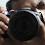1.10
Red 3
Green 2
Blue 4

2.1.I got it too

3.Last line is 4*3=12+2=14

1.NO. last line is 4+3=7 7*2=14

2.NO. You need to to the mutiplication first (BIDMAS - Brackets, Indices, Division, Multiplication, Addition, Subtraction).
See @Jena below

4.4+3×2=4+6=10

5.3*3*3 = 27
2*2*2*3 = 24
3*2*4*4 = 96
4+3*2 = 10